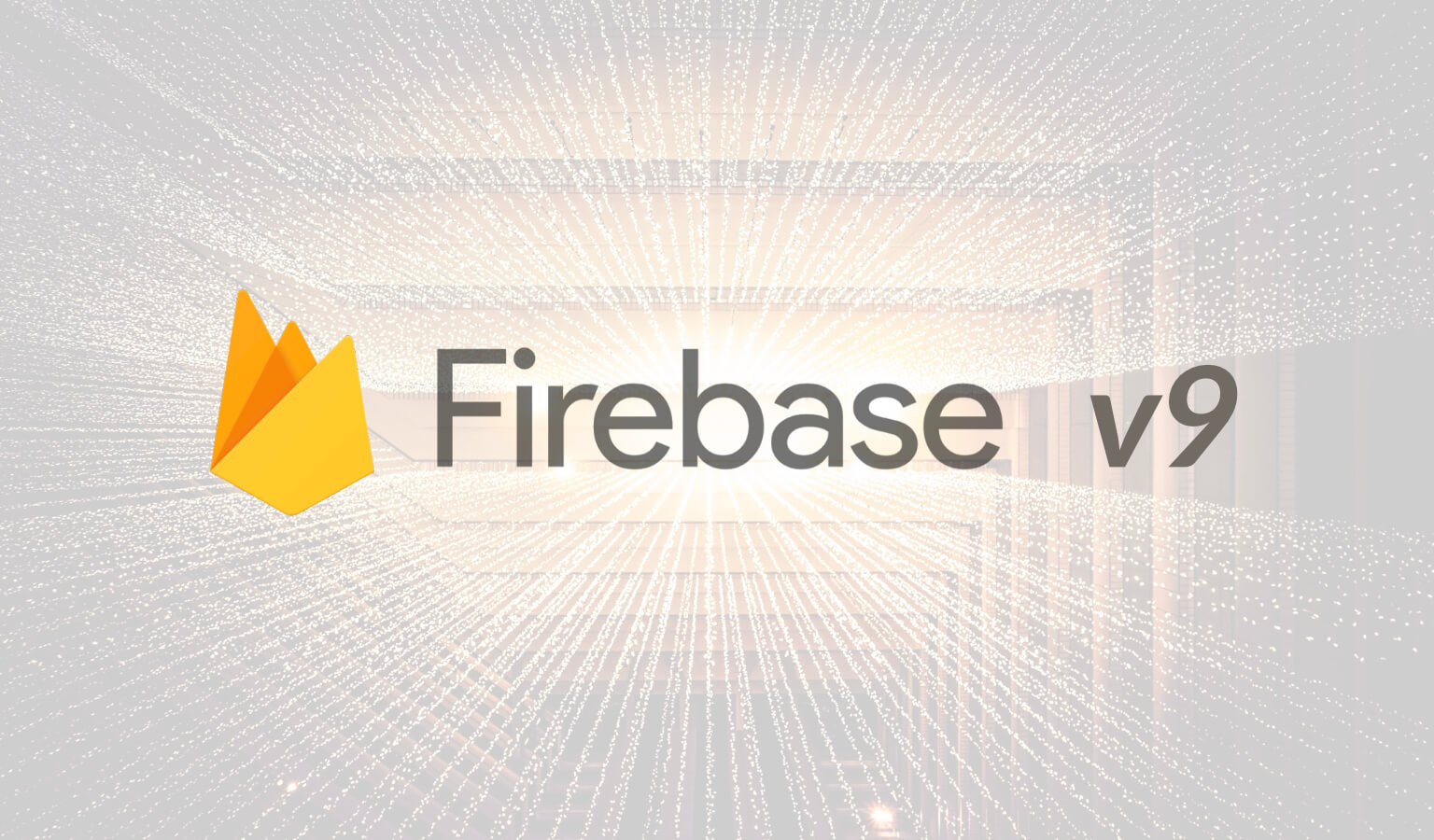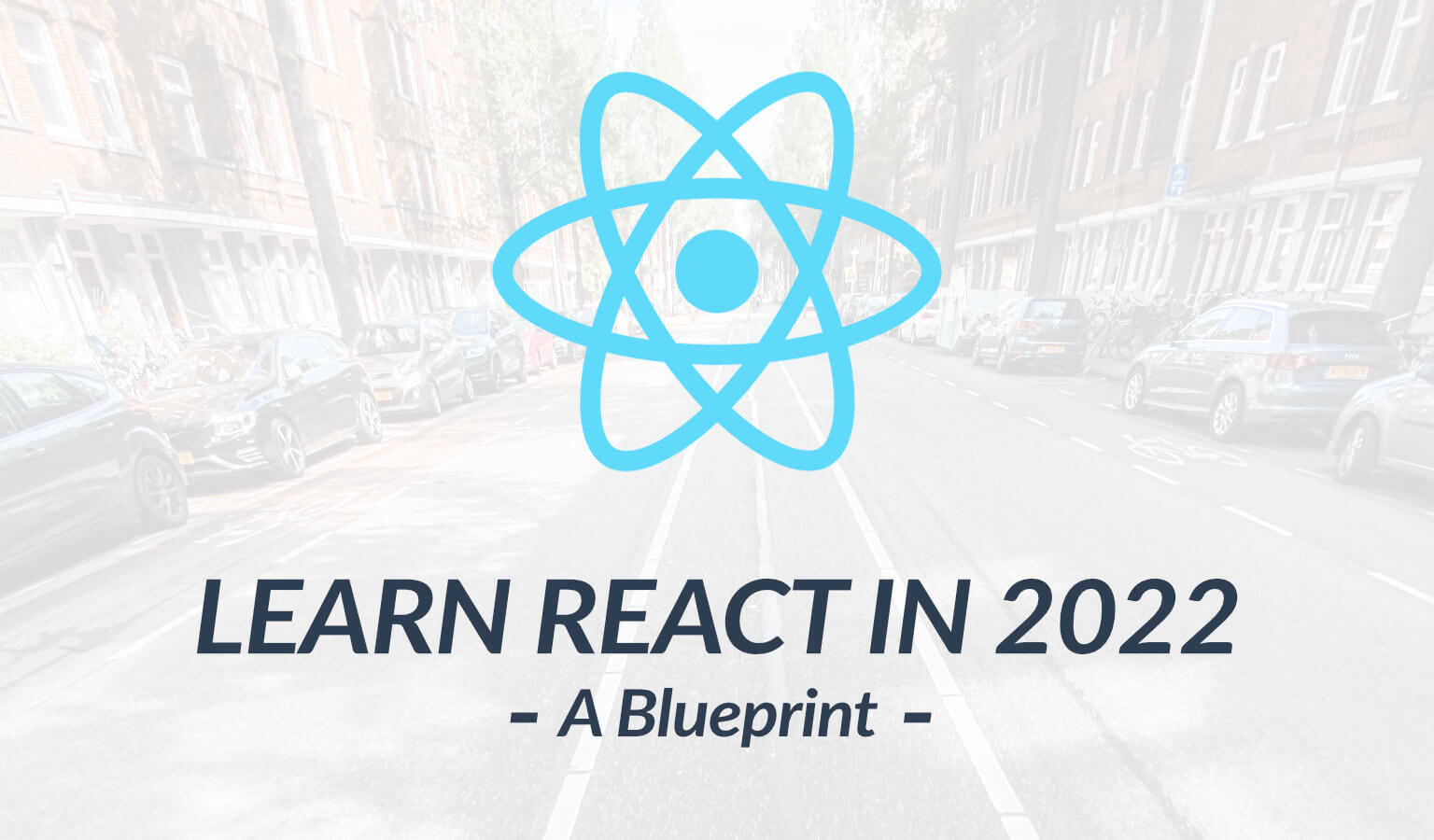# Using Two For Loops In JavaScript: Part 1

So here is an array:

``var array = [3, 2, 3, 5, 5, 7, 5, 1];``

There are times when you will need to loop through this array comparing one number with the other numbers. For instance, you start with 3 and compare it with 3, then 2, then 3, then 5, then 5, etc. until you get to the end.

Next, you may want to move to the second number and cycle through the numbers again. So you would take 2 and compare it with 3, then 2, then 3, then 5, then 5, etc. until you get to the end.

This is very useful and very common. So, how do we do this?

We do this by using two for loops.

### The First Loop

The first loop will cycle us through the numbers. This is just a standard for loop:

``````for(var i = 0; i < array.length; i++){
// do something
}``````

For those unfamiliar with for loops, this loop basically says three things:

1. var i = 0 sets the variable before the loop starts (NOTE: This is a ZERO and not the letter O). So the variable i will equal 0.
2. i < array.length is the "if" statement. If i is less than the length of the array, the loop will execute.
3. i++ says that after the loop does something, the variable i will increment one (0 will become 1).

### The Second Loop

The second loop is going to look the same but with a different variable. Lets make that different variable the letter j:

``````for(var j = 0; j < array.length; j++){
// do something
}``````

The second loop will also cycle through the numbers but there are a few differences.

1. This loop is nested inside the first for loop. This means the first loop will execute, move to the second loop where the second loop will continue to loop until completion, and then the first loop will execute again, move to the second loop where the second loop will loop until completion, etc., etc., until the first loop is completed.
2. The variable is different. This allows the second loop to move through the array independent of the first loop's variable.

### The Two For Loops Together

So here are both loops together:

``````for(var i = 0; i < array.length; i++){
for(var j = 0; j < array.length; j++){
// do something
}
}``````

So lets look at our array again:

``var array = [3, 2, 3, 5, 5, 7, 5, 1];``
• The first loop will start with  which is the number 3 (remember in arrays the first number index is 0).
• The first loop will sit on the number 3 while the second loop, with the variable j, cycles through all the numbers.
• Once all the numbers have been cycled through, the first loop then moves from 0 to 1 (x++), looks at the conditional (is 1 < array.length), and then executes, which looks at the second loop again. The second loop cycles through all the numbers while the first stays on 2. And repeat….

### A Practical Example

So what do two for loops look like practically? Well, we will look at a practical example in Part 2.

----------

About MeTravis of

#### Travis Media

Who Am I? I was 34 years old in a job I hated when I decided to learn to code. Read More
Recent Posts
Explore

### You May Also Like### Navigating S3 Using the AWS SDK for Javascript: A Guide

In this post, we'll look at how to navigate S3 using the AWS SDK for …### How to use Firebase v9 with React (setup, authentication and CRUD examples)

Firebase v9 takes a modular approach which is different from previous …### 5 Steps for Learning React JS in 2022: A Blueprint

Planning on learning React JS in 2022? This React JS learning roadmap …